• 4.8 微分方程微分方程是数值计算中常见的问题，MATLAB提供了多种函数来计算微分方程的解。4.8.1 常微分方程众所周知，对一些典型的常微分方程，能求解出它们的一般表达式，并用初始条件确定表达式中的任意常数。但...


4.8  微分方程微分方程是数值计算中常见的问题，MATLAB提供了多种函数来计算微分方程的解。4.8.1  常微分方程众所周知，对一些典型的常微分方程，能求解出它们的一般表达式，并用初始条件确定表达式中的任意常数。但实际中存在有这种解析解的常微分方程的范围十分狭窄，往往只局限在线性常系数微分方程(含方程组)，以及少数的线性变系数方程。对于更加广泛的、非线性的一般的常微分方程，通常不存在初等函数解析解。由于实际问题求解的需要，求近似的数值解成为了解决问题的主要手段。常见的求数值解的方法有欧拉折线法、阿当姆斯法、龙格-库塔法与吉尔法等。其中由于龙格-库塔法的精度较高，计算量适中，所以使用的较广泛。数值解的最大优点是不受方程类型的限制，即可以求任何形式常微分方程的特解(在解存在的情况下)，但是求出的解只能是数值解。1．龙格-库塔方法简介对于一阶常微分方程的初值问题，在求解未知函数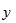时，在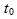点的值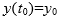是已知的，并且根据高等数学中的中值定理，应有：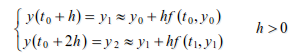一般而言，在任意点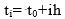，有：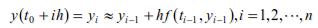当确定后，根据上述递推公式能算出未知函数在点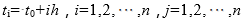的一列数值解：当然在递推的过程中同样存在着一个误差累计的问题，实际计算中的递推公式一般都进行过改造，龙格-库塔公式为：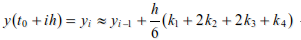其中：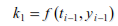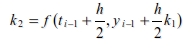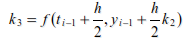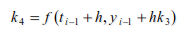2．龙格-库塔法的实现基于龙格-库塔法，MATLAB提供了ode系列函数求常微分方程的数值解。常用的有ode23 和ode45函数，其调用语法如下。(1)[t,y]=ode23(filename,tspan,y0)：采用了二阶、三阶龙格-库塔法进行计算。(2)[t,y]=ode45(filename,tspan,y0)：采用了四阶、五阶龙格-库塔法进行计算。其中filename是定义f(t,y)的函数文件名，该函数文件必须返回一个列向量。tspan的形式为[t0,tf]，表示求解区间。y0是初始状态列向量。t和y分别给出时间向量和相应的状态向量。这两个函数分别采用了二阶、三阶龙格-库塔法和四阶、五阶龙格-库塔法，并采用自适应变步长的求解方法，即当解的变化较慢时采用较大的步长，从而使得计算的速度很快；当解的变化较快时步长会自动地变小，从而使得计算的精度很高。【例4-45】  设有初值问题：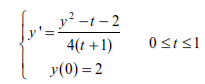试求其数值解，并和精确解相比较，精确解为(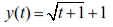)。首先要建立微分方程所对应的函数文件myodefun.m，文件内容如下：function y=myodefun(t,y)                      %  建立函数文件myodefun.my=(y^2-t-2)/(4*(t+1));建立myodefun函数之后，就可以调用ode23函数求解微分方程。>> t0=0;>> tf=10;>> y0=2;>> [t,y]=ode23 ('myodefun',[t0,tf],y0);       %  求数值解>> y1=sqrt(t+1)+1;                              %  求精确解>> plot(t,y,'k.',t,y1,'r')通过图形比较，数值解用黑色圆点表示，精确解用红色实线表示，如图4-13所示。【例4-46】  求下面无劲度系统微分方程组的数值解。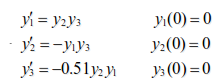为了求解方程，首先要建立方程的m文件。本例中不妨建立名为rigid.m的函数文件，此文件用以描述给出的方程组，文件的内容如下：function dy = rigid(t,y)dy = zeros(3,1);                 %  一个列向量dy(1) = y(2) * y(3);dy(2) = -y(1) * y(3);dy(3) = -0.51 * y(1) * y(2);本例中，我们通过odeset函数对误差进行控制，另外在时间[0 12]进行求解，0时刻初始条件向量为[0 1 1]。>> options =odeset('RelTol',1e-4,'AbsTol',[1e-4 1e-4 1e-5]);%  误差控制>> [T,Y] = ode45(@rigid,[0 12],[0 11],options);               %  求数值解>>plot(T,Y(:,1),'-',T,Y(:,2),'-.',T,Y(:,3),'.')               %  绘制结果图得到的结果如图4-14所示。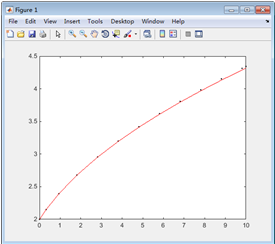图4-13  常微分方程结果图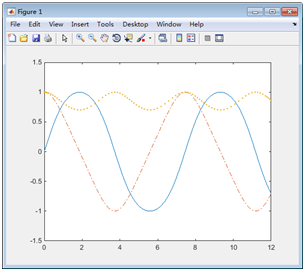图4-14  常微分方程数值展开全文• 利用面积法高阶微分方程系数，此为matlab文件，如有疑问可私信本人。
• 1、根据数学理论常系数线性微分方程可以求解解析解要想解析解可以用dsolve（）函数如果有初始值，则可以在dsolve函数参数funm后边添加初始值参数！2、其他微分方程可以求解数值解一般数值解可以利用ode45（），...
1、根据数学理论常系数线性微分方程可以求解解析解要想求解析解可以用dsolve（）函数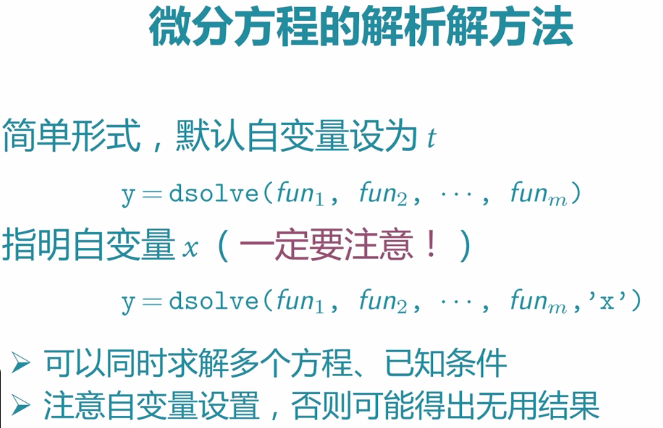如果有初始值，则可以在dsolve函数参数funm后边添加初始值参数！2、其他微分方程可以求解数值解一般求数值解可以利用ode45（），[Tout,Yout]=ode45(fun,[t0,tfinal],x0,option);注释：对于Tout，Yout分别是返回的时间向量和对应的数值解。       fun是对应的微分方程要表示成标准形式（fun函数的描述有三种形式：m文件，匿名函数，inline形式（不建议使用））；      [t0,tfinal]是时间跨度；      x0代表微分方程的初始值；      option是可选选项，他可以用option.RelTol=1e-10(它默认是1e-3)控制误差精度;3、如果是刚性微分方程：  什么是刚性微分方程：一个量变化很小另一个变化却很大 。 刚性微分方程可以用ode15s()这个函数，具体用法跟ode45利用方法一致。
展开全文• 用矩阵函数求解一阶线性常系数齐次微分方程组主要步骤1.问题形式2.矩阵函数3.代入矩阵A的指数函数得最终解 主要步骤 本来想用在矩阵论期中开卷考试验证计算结果的，结果一个解方程组的题也没考…在一些学习网站白...
用矩阵函数求解一阶线性常系数非齐次微分方程组主要步骤1.问题形式2.求矩阵函数3.代入矩阵A的指数函数得最终解
主要步骤
本来想用在矩阵论期中开卷考试验证计算结果的，结果一个解方程组的题也没考…在一些学习网站白嫖惯了，也该分享一下知识，希望这里的code能对搜索到的人有帮助。
主要应用了matlab的符号矩阵，讲解不侧重推导，只侧重编程实现，详情可以看矩阵论相关的参考书。
1.问题形式
一阶线性常系数齐次微分方程组形式为：
$\begin{array}{rl} \frac{\mathrm{d} \xi_{1}}{\mathrm{d} t} & =a_{11} \xi_{1}+a_{12} \xi_{2}+\cdots+a_{1 n} \xi_{n} +\beta_1(t) \\ \\ \frac{\mathrm{d} \xi_{2}}{\mathrm{d} t} & =a_{21} \xi_{1}+a_{22} \xi_{2}+\cdots+a_{2 n} \xi_{n} +\beta_2(t) \\ & \vdots \\ \frac{\mathrm{d} \xi_{n}}{\mathrm{d} t} & =a_{n 1} \xi+a_{n 2} \xi_{2}+\cdots+a_{m} \xi_{n}+\beta_n(t) \end{array}$
将其改写为矩阵方程：
$\boldsymbol{x^{\prime}}=\frac{d\boldsymbol{x}}{d t}=\boldsymbol{Ax}+\boldsymbol{b}(t)$
其一般解为：
$\boldsymbol{x}(t)=\mathbf{e}^{\left(t-t_{0}\right) \boldsymbol{A}} \boldsymbol{x}_{0}+\mathrm{e}^{t\boldsymbol{A}} \int_{t_{0}}^{t} \mathrm{e}^{-s\boldsymbol{A}} \boldsymbol{b}(s) \mathrm{d} s$
其中$\boldsymbol{x}_0$为变量的初始值。
2.求矩阵函数
矩阵函数$e^{t\boldsymbol{A}}$的求解采用若当标准型法。
代码如下：
定义函数，输出为矩阵函数的矩阵
function [EtA,P,J] = my_expm( A )

首先求若当标准型，这里注意一定要将矩阵$A$转化为符号矩阵形式，标准型和特征向量会以分数和根号形式表示，并将所有若当块用元组（cell）存储
syms t x%x表示奇异值
[P,J] = jordan(sym(A));%先求若当标准型
n = size(A,1);
J_ = {};
%num存储若当块的个数
num = 1;pre = 1;
for i = 2:n
if J(i-1,i) == 0
J_{num} = J(pre:i-1,pre:i-1);
pre = i;
num = num + 1;
if i == n
J_{num} = J(pre,pre);
end
end
if i == n && J(i-1,i) == 1
J_{num} = J(pre:n,pre:n);
end
end

搜索尺寸最大的若当块，因为特征多项式的导数次数由最大的若当块尺寸决定，这个尺寸可以和上一次遍历合并，这样一次遍历就可以得到所有的若当块和最大若当块尺寸。
%搜索最大维度
mSize = 0;
for i = 1:num
if size(J_{i},1) > mSize
mSize = size(J_{i},1);
end
end

在这里定义特征多项式和其$n-1$次导数除以$(n-1)!$的形式，存入数组备用。
%定义矩阵多项式的导数
f_J = [exp(t*x)];
for j = 1:mSize-1
f_J = [f_J,diff(exp(t*x),x,j)/prod(1:j)];
end
f_J = simplify(f_J);

对每一个若当块求特征函数矩阵，将所有特征函数矩阵对角合并到一起。
EtJ = [];
%blkdiag对角合并
for i = 1:num%若当块索引
n1 = size(J_{i},1);
etJ = subs(f_J(1),x,J_{i}(1,1))*diag(ones(1,n1));
if n1 >= 2
for j = 1:n1-1%若当块维度索引
etJ = etJ + diag(subs(f_J(j+1),x,J_{i}(1,1))*ones(1,n1-j),j);
end
end
EtJ = blkdiag(EtJ,etJ);%若当块合并
end
EtJ = simplify(EtJ);
EtA = P*EtJ*(inv(P));

输入：
$A=\left[\begin{array}{rrr} -2 & 1 & 0 \\ -4 & 2 & 0 \\ 1 & 0 & 1 \end{array}\right]$
输出：
ans =
[          1 - 2*t,              t,      0]
[             -4*t,        2*t + 1,      0]
[ 2*t - exp(t) + 1, exp(t) - t - 1, exp(t)]

3.代入矩阵A的指数函数得最终解
定义函数
function [x,EtA] = my_solve_equation( A,b,co,to )
%输入：A系数矩阵，b非齐次项（齐次为0）,co初始值,to初始时刻
%一阶线性常系数非齐次\齐次微分方程组

将$t$替换为$t-t_0$求解解得第一项
%先求矩阵A指数函数
[EtA,~,~] = my_expm( A,1 );
syms t s
Firs = subs(EtA,t,t-to)*co;

求解第二项矩阵积分项
b = subs(b,t,s);%一步符号替换
E_tA = subs(EtA,t,-s);
Secn = EtA*int(E_tA*b,s,to,t);
x = simplify(Firs + Secn);

输入：
$\boldsymbol{A}=\left[\begin{array}{rrr} -2 & 1 & 0 \\ -4 & 2 & 0 \\ 1 & 0 & 1 \end{array}\right]\quad \boldsymbol{b}(t)=\left[\begin{array}{rrr} 1\\ 2\\ e^{t}-1 \end{array}\right]\quad \boldsymbol{x}(0)=\left[\begin{array}{rrr} 1\\ 1\\ -1 \end{array}\right]\quad t_0 = 0$
输出：
ans =
1
1
exp(t)*(t - 1)



展开全文算法
• 本文主要对简单的连续系统微分方程（含系数矩阵）的MATLAB数值解法进行归纳，并绘制仿真图像。同时也对一阶和高阶微分方程求法进行总结。 系统方程为一阶积分器的连续系统 目录1. 可解析解的微分方程2. MATLAB...

在多智能体系统（MASs）论文数值仿真时，时常碰到连续系统的情况，其中参数也多为系数矩阵。本文主要对简单的连续系统微分方程（含系数矩阵）的MATLAB数值解求法进行归纳，并绘制仿真图像。同时也对一阶和高阶微分方程求法进行总结。

目录1. 可求解析解的微分方程2. MATLAB函数直接调用3. 其他情形3.1 一阶微分方程3.2 高阶微分方程4. 总结参考资料
1. 可求解析解的微分方程
系统方程为一阶积分器的连续系统 $\dot{x}=u,~~x \in R^n,$
其中为了实现平均一致性，设计控制器  $u=-Lx$, $L \in R^{n \times n}$ 为对应图的拉普拉斯矩阵。
为了进行系统仿真,设 $n=4,L$ 如下，此处连续系统方程为
$\dot{x}==-\begin{pmatrix} 3 & -1 & -1 & -1 \\ -1 & 2 & -1 & 0 \\ -1 & -1 & 3 & -1 \\ -1 & 0 & -1 &2 \\ \end{pmatrix}x$
假设初值 $x_0=(10;0;5;10)$， 对其进行数值求解与仿真。
可见对于如上 $\dot{x}=-Lx$ 形式的微分方程，可用常微分方程的知识，先求出其解析解，再进行数值仿真。解析解为：
$x=e^{-Lt}x_0.$
MATLAB代码如下：
%%初始化设置
step=200;          %定义迭代步数
y=zeros(step,4);   %初始化矩阵来存储迭代值，用于作图
x0=[10; 0; 5; 10]; %微分方程初值
L=[3 -1 -1 -1;     %系数矩阵L
-1  2 -1  0;
-1 -1  3 -1;
-1  0 -1  2];
for i=1:4         %迭代初值
y(1,i)=x(i);
end

%%系统迭代步
for k=2:step      %迭代过程
x=expm(-L*k/50)*x0;   %注意指数矩阵求解用函数expm()
for i=1:4
y(k,i)=x(i);
end
k=k+1;
end

%%仿真作图
t=1:step;
plot(t/50,y(t,1),t/50,y(t,2),t/50,y(t,3),t/50,y(t,4))
xlabel('t');
ylabel('x');
legend('x1','x2','x3','x4')

仿真图像如下：2. MATLAB函数直接调用
在很多情况下，微分方程的解析解是很难求得的，所以才需要求其数值解并进行仿真。这里主要运用MATLAB自带函数ODE45（龙格库塔方法）来求解。
对于题设 1 中问题，我们将其转化为微分方程组，如下：
$\left\{ \begin{array}{c} \dot{x_1}=-[~ 3x_1-x_2-x_3-x_4~] \\ \dot{x_2}=-[~ -x_1+2x_2 -x_3+0~]\\ \dot{x_3}=-[~ -x_1-x_2+3x_3-x_4~] \\ \dot{x_4}=-[~ -x_1+0-x_3+2x_4~] \end{array} \right.$
定义m文件 $func.m$，将函数信息存入此m文件。
function dx=func(t,x)

%% 初始化有4个分量的dx
dx=zeros(4,1);

%% 微分方程
dx(1)=-(3*x(1)-x(2)-x(3)-x(4));    %dx(1)为x第一个分量的导数，下同
dx(2)=-(-x(1)+2*x(2)-x(3)+0);      %x(1)指x的第一个分量，下同
dx(3)=-(-x(1)-x(2)+3*x(3)-x(4));
dx(4)=-(-x(1)+0-x(3)+2*x(4));

如下代码对函数进行调用，并求数值解与仿真。
%% 参数初始化
startt=0;endd=10;      %区间开始和结束
x1=10;x2=0;x3=5;x4=10; %变量初值

%% ode45方法求解数值解
[t,x]=ode45(@func,[startt endd],[x1;x2;x3;x4]);

%% 仿真图像
plot(t,x(:,1),t,x(:,2),t,x(:,3),t,x(:,4))
xlabel('t');
ylabel('x');
legend('x1','x2','x3','x4')

仿真图像绘制如下：3. 其他情形
3.1 一阶微分方程
简单的一阶微分方程，无需将微分方程写入m文件，只需在命令行的ODE45函数中加入微分方程即可。例如求解如下微分方程数值解
$\dot{x}=x+t,$
MATLAB代码如下：
%% 定义x初值
x0=0;
%% ode5求微分方程数值解，其中[0 6]为求解区间
% @(t,x) x+t 为要求解的微分方程表示
[t,x]=ode45(@(t,x) x+t,[0 6],x0);
%% 绘制图像
plot(t,x);

仿真图像显示如下：3.2 高阶微分方程
高阶微分方程求数值解，基本思路是将其化为一阶微分方程组进行求解。例如对于如下二阶微分方程：
$\ddot{x}+x^3+\dot{x}^3=0,\\ x(0)=1,~\dot{x}(0)=0.$
我们将其化为两个一阶微分方程，如下：
$\dot{x}_1=x_2,\\ \dot{x}_2=-{x_1}^3-{x_2}^3.$
其实，$x_1$表示原系统$x$,  $x_2$表示原系统 $\dot{x}$. 化为一阶方程组后，便可采用 2 中介绍的方法，先定义M文件 $funcc.m$ 存储如上微分方程信息。MATLAB代码如下：
function dx=funcc(t,x)
dx=[x(2);
-x(1)^3-x(2)^3];


命令行输入如下代码，调用m文件，并求解与绘图。
%% 给定x1和x2初值
x0=[1;0];

%% 使用ode45求解微分方程
[t,x]=ode45(@funcc,[0 20],x0);

%% 仿真作图（分别绘制了x和x的导函数图像）
plot(t,x(:,1),t,x(:,2))
xlabel('t');
ylabel('x');
legend('x_1','x_2')

仿真图像如下所示：4. 总结

微分方程数值解求法总结：

直接求出其解析解，便可计算其数值解，如情况1。
不可求解析解的形式：
2.1 一阶微分方程，如情况3.1。
2.2 一阶微分方程组，如情况2。
2.3 高阶微分方程，如情况3.2。

参考资料

  https://blog.csdn.net/qq_18820125/article/details/104727013
 https://www.zhihu.com/question/22378594


展开全文ieee论文 矩阵
• 线性常微分方程的解满足叠加原理,从而他们的求解可归结为一个特解和相应齐次微分方程的通解.一阶变系数线性微分方程总可用这一思路求得显式解。高阶线性常系数微分方程可用特征根法求得相应齐次微分方程的基本解，...
• dsolve函数是matlab中自带的可以用来解决部分常微分方程的函数（方法），下面以物理学中的热传导问题为例展示如何使用这个函数。 热力学模型假设物体和恒温的环境接触，温度随时间的变化率和温差成正比，我们假设...
• 不确定是否正确，希望有大佬能够更明确的指出其中的错误，作出指导)对于刚性和非刚性微分方程的区分，可以简单的转变为在将原方程转换为常微分方程组后，进行一个简单的系数判断：例如：y‘‘‘ - 3y‘‘ - y‘y = 0...
• 引入首先用高数知识求解非齐次常系数微分方程image.png再利用信号与系统中冲激响应求解验证image.png利用MATLAB求解验证y=dsolve('D2y+3*Dy+2*y=exp(-t)','y(0)=1','Dy(0)=2','t')得出结果：y =(t - 2 exp(-t) + 3) ...
• 引入首先用高数知识求解非齐次常系数微分方程image.png再利用信号与系统中冲激响应求解验证image.png利用MATLAB求解验证y=dsolve('D2y+3*Dy+2*y=exp(-t)','y(0)=1','Dy(0)=2','t')得出结果：y =(t - 2 exp(-t) + 3) ...
• 首先用高数知识求解非齐次常系数微分方程 再利用信号与系统中冲激响应求解验证 利用MATLAB求解验证 y=dsolve('D2y+3*Dy+2*y=exp(-t)','y(0)=1','Dy(0)=2','t') 得出结果： y = (t - 2 exp(-t) + 3) exp(-t) ...python
• 一般解微分方程组都是交给计算机的任务，这里直接使用matlab中的eig（）函数出，函数用法为：[λ,V]=eig(A); 此时，在matlab中，V为：由特征向量为每一列组成的五阶方阵，λ是：仅有以特征值为对角线元素构成矩阵 线性代数
• 1. 曲线长度积分公式 2. 已知曲线方程 3. 已知系数，曲线方程为 y = 0.0001 * x³ + 0.0005 * x² + 20 ...5. matlab中定义曲线方程 ...6. matlab中求解曲线方程微分 >> z = diff(y,x) z = (...
• 题目描述：某系统满足的微分方程为$y^{''}(t)+4y^{'}(t)+3y(t)=2f^{'}(t)+f(t)$，系统的单位冲击响应. impulse函数 impulse函数可以求得系统的单位冲击响应，参数为sys和t，其中sys为系统对应的微分方程，t为...
• ## MATLAB常用算法

热门讨论 2010-04-05 10:34:28
DEYCJZ_ myds 用修正的亚当斯预测校正法一阶常微分方程的数值解 DEYCJZ_hm 用汉明预测校正法一阶常微分方程的数值解 DEWT 用外推法一阶常微分方程的数值解 DEWT_glg 用格拉格外推法一阶常微分方程的数值解 ...
• DEYCJZ_ myds 用修正的亚当斯预测校正法一阶常微分方程的数值解 DEYCJZ_hm 用汉明预测校正法一阶常微分方程的数值解 DEWT 用外推法一阶常微分方程的数值解 DEWT_glg 用格拉格外推法一阶常微分方程的数值解 ...
• 微分方程微分方程 多项式 Matlab提供了一组函数用于处理多项式运算。 （不适合处理大于10的高阶多项式） 常用的函数：roots, polyval, polyfit, … %%多项式表达： % 用一个行向量表示各阶系数，阶次降序排列。...
• MATLAB中求解常系数微分方程可以利用dsolve(‘eq1,eq2,…’,’cond1,cond2,…’,’v’)函数，其中参数eq表示各个微分方程，微分和求导的输入是使用Dy，D2y，D3y来表示yed一阶导数、二阶导数和三阶导数；...
• Matlab求解系统的响应 LTI系统的零状态响应的函数lsim，其调用格式为 y = lsim(sys,f,t) 式中，t表示计算系统响应的抽样点向量；f是系统输入信号，sys...式中，b和a分别为微分方程右端和左端各项的系数。 如 ...
• 1、tf(b,a) 根据微分方程求转移函数  a，b为分子分母多项式系数或者说微分方程左边右边的系数   2、tfestimate()  [Txy,F] = tfestimate(x,y,window,noverlap,nfft,fs)  根据输入输出，估计频率响应函数 ...数字信号处理 常用函数
• ## matlab求解系统响应

千次阅读 2020-07-29 19:22:32
% sys 需要借助tf函数获得 ,sys = tf(b,a) ： b,a分别是微分方程右端和左端各项的系数 % 例题：y''(t)+ 2y'(t)+ 77y(t) = f(t) 在t>=0时接入激励f(t)=10*sin(2 *pi *t),零状态响应 a = [ 1 2 77]; b = ; sys...
• 第11章 积分和微分方程组 151 11.1 积分 151 11.2 常微分方程组 154 第12章 MATLAB程序设计 165 12.1 条件控制语句 165 12.2 循环语句 168 12.3 M文件的其他相关内容 176 12.4 将函数作为参数传递给其他函数 186 ...
• 二、方程根；三、线性方程式和线性系统；四、统计；五、回归与内插 一、多项式/数值微积分 1 多项式 1.1 表示：行向量a（表示多项式的系数） 1.2 polyval(a,x); %x是自变量 2 多项式微分 2.1 polyder(a)...
• 面积法的原理是是通过微分方程系数来辨识传递函数：首先求得放大系数，得到无因次阶跃响应y(t)大多数自平衡对象的y(t)可近似为 an*dny(t)/dt+an-1 *dn-1y(t)/dt+······+a1*dy(t)/dt+y(t)=u(t)现以三阶...
• 四点法得到的代数矩阵为三对角线性方程组AU=b，一般可以用matlab求逆命令求解U，但因本题中系数矩阵规模较大，且主对角线和两个次对角线之外，其余元素均为0，因而求逆解方程组会导致浪费内存、程序运行缓慢。...数学建模 算法
• 第9～15 章为工程应用部分，讲解偏微分方程、优化、图像处理、信号处理、小波分析等工具箱，Simulink 仿真基础及应用等；第16～20 章为知识拓展部分，讲解句柄图形对象、图形用户界面、文件读取I/O、MATLAB 编译器和...
• 9．1 偏微分方程（PDE）工具箱介绍 9．1．1 偏微分方程常见类型介绍 9．1．2 偏微分方程工具箱简介 9．2 求解偏微分方程示例 9．2．1 求解椭圆方程 9．2．2 求解抛物线方程 9．2．3 求解双曲线方程 9．2．4 求解特征...# matlab求微分方程系数matlab 订阅